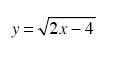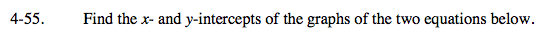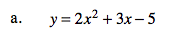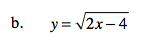### Home > A2C > Chapter 4 > Lesson 4.1.4 > Problem4-55

4-55.
1. Find the x- and y-intercepts of the graphs of the two equations below. Homework Help ✎

1. y = 2x2 + 3x − 5

2.To find the x-intercept, y = ?
To find the y-intercept, x = ?x-intercepts: (2, 0)

y-intercepts: none